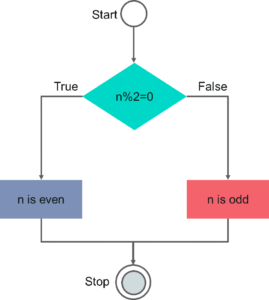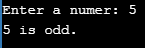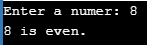# How to Implement Odd & Even Program in C

Published on Sep 11,2019 2.2K ViewsChecking whether a number is even or odd, is a very common problem in C language. Following pointers will be covered in this Odd & Even Program in C article:

If a number is perfectly divisible by 2 it is known as even number. The remaining numbers which are not perfectly divisible by 2 is known as odd number. In simple words, even numbers are those numbers which are in form n = 2k, whereas odd numbers are those numbers which are in form of n = 2k+1. All the integers will either be even numbers or odd numbers. In this blog we will understand how to check whether a number is even or odd using C program.

There are various ways in which we can check whether a given number is even or odd.

Let’s look at each one of them one by one.

## Odd & Even Program Flow Diagram

Following would be the flow diagram for the odd or even program:Now let us look at the algorithm for odd & even Program in C.

## Odd & Even Program Algorithm

The algorithm is very simple:

START

Step 1 → Take a integer n

Step 2 → Assign n to the variable

Step 3 → Perform n modulo 2 and check result if output is 0

Step 4 → If true n is even

Step 5 → If false n is odd

STOP

Now let us look at the algorithm for Odd & Even Program Pseudocode

## Odd & Even Program Pseudocode

IF (integer modulo 2) equals to 0

PRINT number is even

ELSE

PRINT number is odd

END IF

Now let us look at the algorithm for Program to Check Even or Odd

## Program to Check Even or Odd

In C programming language, we have a modulo operator which returns the remainder of the divisor & divident. We will use this operator to figure out whether the nuber is 2k format or 2k+1 format.

Now, let ius look at the code to check whether a given integer is odd or even.

Code

```if(num % 2 == 0)
printf("%d is even.", num);
else
printf("%d is odd.", num);

```

Moving ahead, let us look at the complete code.

Example

```#include <stdio.h>
int main()
{
int num;
printf("Enter a numer: ");
scanf("%d", &num);
if(num % 2 == 0)
printf("%d is even.", num);
else
printf("%d is odd.", num);
return 0;
}

```

Output1:Output2:In this program we checked whether the given number is returning 0 or 1 remainder when we divide it with 2. If n%2==0, the number is even, else the number is odd.

Now let’s look at one more variation where you can perform the same.

## Program to Check Odd or Even Using Conditional Operator

You can also use conditional operator to verify the same condition which we have discussed earlier.

Conditional Operator/Ternary operator: Conditional operators return one value if condition is true and returns another value is condition is false.

Syntax     :        (Condition? true_value: false_value);

Example :         (X > 10  ?  0  :  1);

Example

```#include <stdio.h>
int main()
{
int num;
printf("Enter a numer: ");
scanf("%d", &num);
(num % 2 == 0) ? printf("%d is even.", num) : printf("%d is odd.", num);
return 0;
}

```

Output1:Output2:Let us look at one more variation

## Find Odd or Even Using Bitwise Operator

You can also check whether the given number is even or odd using bitwise AND operator.

Example

```#include <stdio.h>
int main()
{
int num;
printf("Enter a numer: ");
scanf("%d", &num);
if (num & 1 == 1)
printf("%d is odd.", num);
else
printf("%d is even.", num);
return 0;
}

```

Output1:Output2:Now after going through the above programs you would have understood how to check whether a given number is odd or even in C programming. I hope this blog is informative and added value to you.

With this, we come to an end of this Odd & Even Program in C article.

check out the training provided by Edureka on many technologies like Java, Spring and  many more, a trusted online learning company with a network of more than 250,000 satisfied learners spread across the globe

Got a question for us? Mention it in the comments section of this “Odd & Even Program in C” blog and we will get back to you as soon as possible.